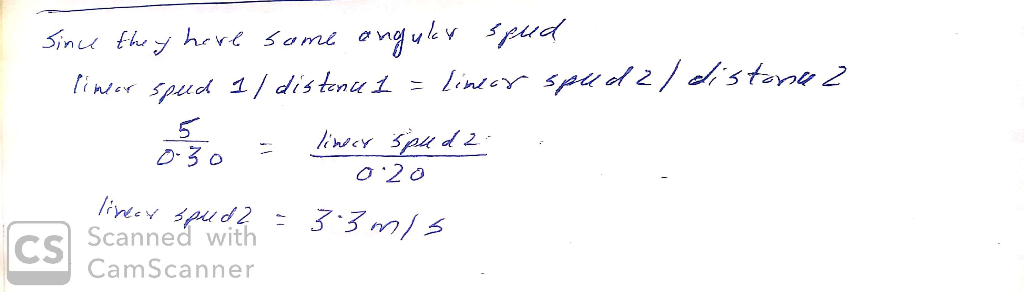Homework Help Question & Answers

# The scale of a spring balance that reads from 0 to 20.9 kg is 20.7 cm long. A package suspended from the balance is found to oscillate vertically with a frequency of 1.60 Hz. (a) What is the spring co...

The scale of a spring balance that reads from 0 to 20.9 kg is 20.7 cm long. A package suspended from the balance is found to oscillate vertically with a frequency of 1.60 Hz. (a) What is the spring constant? (b) How much does the package weigh?##### Add Answer of: The scale of a spring balance that reads from 0 to 20.9 kg is 20.7 cm long. A package suspended from the balance is found to oscillate vertically with a frequency of 1.60 Hz. (a) What is the spring co...
More Homework Help Questions Additional questions in this topic.

• #### A 77-kg man standing on a scale in an elevator notes that as the elevator rises, the scale reads...

Need Online Homework Help?

Get FREE EXPERT Answers
WITHIN MINUTES
Related Questions